An Introduction to Probability

Key Terms

o         Fair

o         Random

o         Probability

o         Chance

o         (Selection) with replacement

o         (Selection) without replacement

Objectives

o         Define and recognize some basic terms associated with probability

o         Calculate probabilities in very simple cases

o         Calculate probabilities for simple cases of successive selection with and without replacement

Probability

You may already be quite familiar with the idea of flipping a coin to make an impartial choice between two alternatives. An average coin is generally assumed to be fair, meaning that it has the same chance of landing heads up as it does of landing tails up. Much of what we have studied thus far can help us to quantify probability and calculate the probabilities of certain events (such as the probability that a coin will land heads up).

We often use terms like "chance," "probability," and "random" without having clear definitions of them in mind. For our purposes, we define the outcome of an event (such as the flipping of a coin) as random if we cannot predict that outcome based on any conditions prior to the event. For instance, when a coin is flipped, we cannot (easily, anyhow) figure out whether it will land heads up or tails up by analyzing the situation (such as how the coin is flipped, the characteristics of the surface on which it lands, and so on).

If we flip a fair coin a large number of times, we find that about half the time, the coin lands heads up. The rest of the time, it lands tails up. Ideally, if we were to perform an infinite number of flips, we would find that the coin landed heads up once out of every two flips on average. This average result is the probability (or chance) of the outcome (heads). Another way of looking at probability is by way of a ratio (or proportion): the probability (in this case) of the coin landing heads up is the total number of "successful" outcomes (one: heads) divided by the total number of possible outcomes (two: heads and tails). We use the notation P(x) to describe the probability of x. Thus,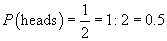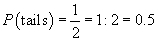Note that the number of successful outcomes can never exceed the total number of possible outcomes--thus, the probability of a particular event (using the ratio format we use above) must always be between zero and one, inclusive. For instance, we might ask what the probability that a coin lands either heads up or tails up. The total number of successful outcomes is two (heads and tails), and the total number of possible outcomes is also two (heads or tails). The probability of this outcome is one: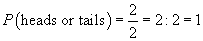Let's consider probability in a slightly more complicated situation. Imagine a standard deck of 52 playing cards. If the deck is shuffled and a random card is chosen (remember that "random" means that we cannot predict the outcome ahead of time), then we can use the preceding principle of probability to calculate the chance that the chosen card is (for instance) the jack of spades. First, we note that the deck contains 52 distinct cards, only one of which is the jack of spades. Thus, the number of successful outcomes of the choice is one, and the total number of possible outcomes is 52. The ratio of successful outcomes to possible outcomes is the probability that a random selection will turn up the jack of spades: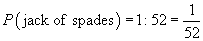To use this approach to calculate a probability, each possible outcome must have the same chance of occurring as does any other possible outcome. (For instance, if a flipped coin is "loaded" such that heads is more likely than tails, we cannot use this approach to calculate probability.) Calculating probabilities in cases where the possible outcomes are not equally likely is possible, but these cases are beyond the scope of this article.

The following practice problems provide you the opportunity to practice calculating probabilities for some other simple cases.

Practice Problem: What is the probability that the roll of a fair six-sided die (a dies with six faces numbered one through six) will turn up an odd number?

Solution: First, note that the die is identified as "fair"; in other words, each possible outcome is just as likely as any other outcome. To calculate a probability, we must calculate the ratio of successful outcomes (odd numbers) to the total number of possible outcomes. Since the die has sides marked 1, 2, 3, 4, 5, 6, we can see that a roll has six potential outcomes. Of these, three are odd numbers (1, 3, and 5). Let's calculate the probability:

Interested in learning more? Why not take an online Pre-Algebra course?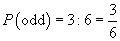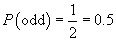Thus, the probability of rolling an odd number is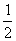, or, in decimal form, 0.5.

Practice Problem: What is the probability of drawing an ace from a standard 52-card deck of playing cards?

Solution: In this case, we are not trying to find the probability of drawing just one card, but of drawing one of several possible cards. A standard deck contains four aces (hearts, spades, diamonds, and clubs); thus, our choice has four possible successful outcomes. Because the deck contains 52 cards, there are 52 possible outcomes (successful and unsuccessful). We can now calculate the probability through the ratio (proportion) of successful outcomes to total possible outcomes: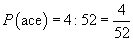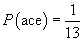Thus, we have a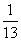chance of picking an ace in a random drawing from a standard deck.

More on Probability-Successive Selections

The cases we considered above are simple and provide a foundation in probability, but we need slightly more information before we can calculate such things as the probability of winning a lottery, for instance. We now consider a more general situation: making multiple successive random choices.

First, we must always specify whether a series of random selections is made with replacement or without replacement. For instance, if we were to choose a card from a deck, replace the card, then make another choice, we would be choosing two cards with replacement. If we were to choose a card and then choose another card from the remaining deck, we would be choosing without replacement. Likewise, if we were to roll a die twice, we would effectively be making two rolls with replacement (because the die has the same six possible outcomes in each case).

Let's consider a fair six-sided die, and let's say we want to calculate the probability of rolling a one followed by a roll of six. We know how to calculate the probability of a roll of a one (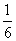) as well as a roll of a six (), but how shall we calculate the probability of a roll of a one followed by a roll of a six? Let's take a look at all the possible outcomes (listed below as the first roll followed by the second roll):

1 - 1    2 - 1    3 - 1    4 - 1    5 - 1    6 - 1

1 - 2    2 - 2    3 - 2    4 - 2    5 - 2    6 - 2

1 - 3    2 - 3    3 - 3    4 - 3    5 - 3    6 - 3

1 - 4    2 - 4    3 - 4    4 - 4    5 - 4    6 - 4

1 - 5    2 - 5    3 - 5    4 - 5    5 - 5    6 - 5

1 - 6    2 - 6    3 - 6    4 - 6    5 - 6    6 - 6

If we count the total number of possible outcomes, we find that there are 36, only one of which is a successful outcome (1 - 6). Thus, the probability is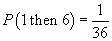Another way of looking at this, though, is that for each potential outcome (six total) of the first roll, there are six possible next rolls. (For a roll of 1, we can then roll 1, 2, 3, 4, 5, or 6; the same applies to a first roll of 2, 3, 4, 5, and 6.) The total number of possible outcomes is the product of the possible outcomes for each successive roll (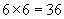). Thus, the probability of getting a certain outcome of a first selection followed by a certain outcome of a second selection is simply the product of the two individual probabilities: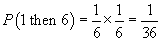Let's consider another example: assume a bag contains three red marbles and one blue marble. We can calculate the probability of selecting two red marbles (without replacement) as follows. In the first selection, the bag contains three red marbles and one blue marble, meaning we have a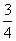chance of selecting a red marble. Assume we select a red marble; then, because we do not replace the marble after the first selection, the bag contains two red marbles and one blue marble. The probability of choosing a red marble in this case is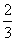. We can calculate the probability of choosing two red marbles (without replacement) by multiplying these individual probabilities: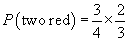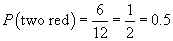Thus, we have a probability of 0.5 of choosing two red marbles without replacement.

Practice Problem: Given a fair coin, what is the probability of the coin yielding four heads in four successive flips?

Solution: In this case, we are making "selections" with replacement (the coin has the same two possible outcomes in each flip). One approach to this problem is to list all the possible outcomes of four flips (below, we use T to represent tails and H to represent heads):

HHHH HHHT HHTT HTTT TTTT

HHTH HTHT THTT

HTHH THHT TTHT

THHH HTTH TTTH

THTH

TTHH

As we can see, out of 16 possible outcomes, only one (HHHH) is "successful." Thus, the probability is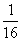. We can also calculate the probability as the product of the individual probabilities of each flip: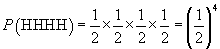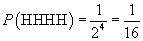This second approach is obviously less time consuming, because we do not need to count large numbers of possibilities. Each approach, however, yields the same result.

Practice Problem: A bag contains five \$1 bills and two \$100 bills. A contestant is allowed to keep his first two choices from the bag. What is the probability that he gets to keep \$200?

Solution: In this case, the contestant is keeping whichever two bills he chooses, so the choices are made without replacement. To get \$200, obviously the contestant must pick a \$100 bill in each of his two successive selections. In the first choice, he has a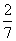chance of picking a \$100 bill. If successful, he then has achance of picking another \$100 bill. The chance of picking both \$100 bills is then the following: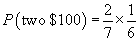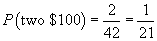The chances of picking both \$100 bills (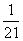) do not look too great!